## Method

Submitted on 16 Aug. 2020 15:23 by
Lin Bai (Worcester Polytechnic Institute)

 Running time: 8m s Environment: GPU @ 2.5 Ghz (Python)

 Method Description: two branches with spatial and context information respectively Parameters: Amda: \alpha=1e-3 reduce on plateau early stopping Latex Bibtex:

## Evaluation in Bird's Eye View

 Benchmark MaxF AP PRE REC FPR FNR UM_ROAD 91.99 % 92.54 % 92.75 % 91.24 % 3.25 % 8.76 % UMM_ROAD 93.98 % 95.19 % 94.47 % 93.49 % 6.01 % 6.51 % UU_ROAD 90.79 % 91.67 % 91.79 % 89.80 % 2.62 % 10.20 % URBAN_ROAD 92.55 % 93.21 % 92.94 % 92.16 % 3.86 % 7.84 %
This table as LaTeX

## Behavior Evaluation

 Benchmark PRE-20 F1-20 HR-20 PRE-30 F1-30 HR-30 PRE-40 F1-40 HR-40
This table as LaTeX

The following plots show precision/recall curves for the bird's eye view evaluation.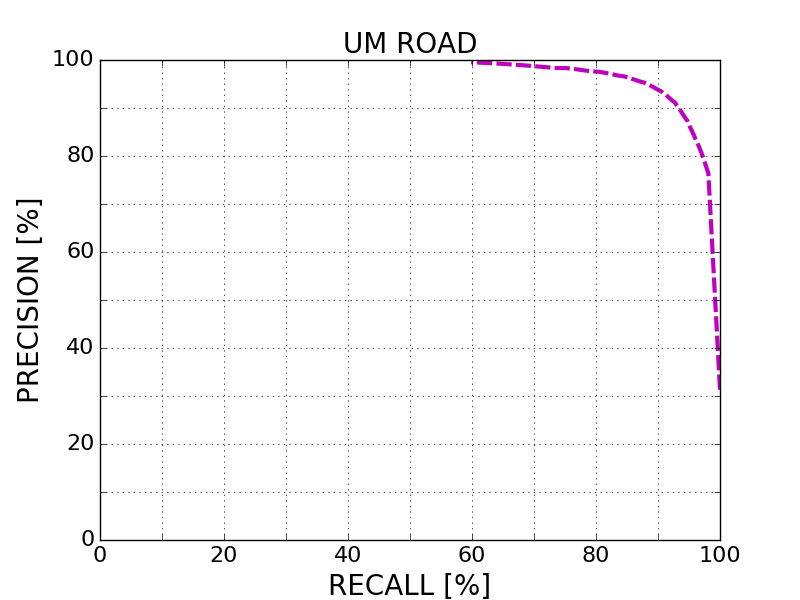This figure as: png eps pdfThis figure as: png eps pdfThis figure as: png eps pdfThis figure as: png eps pdf

## Distance-dependent Behavior Evaluation

The following plots show the F1 score/Precision/Hitrate with respect to the longitudinal distance which has been used for evaluation.

## Visualization of Results

The following images illustrate the performance of the method qualitatively on a couple of test images. We first show results in the perspective image, followed by evaluation in bird's eye view. Here, red denotes false negatives, blue areas correspond to false positives and green represents true positives.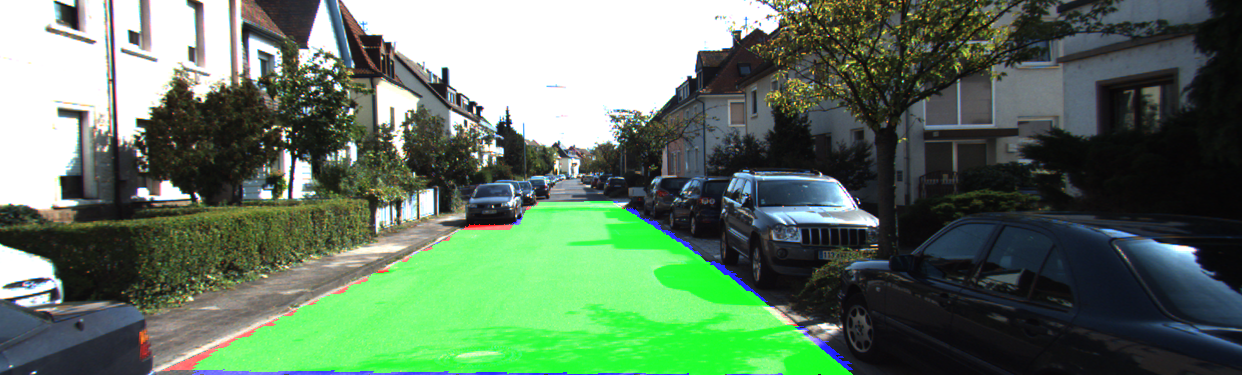This figure as: png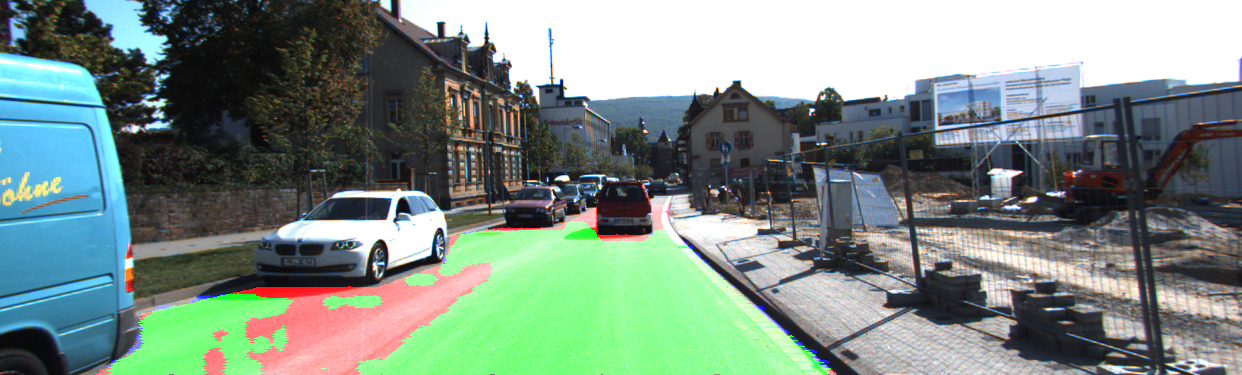This figure as: png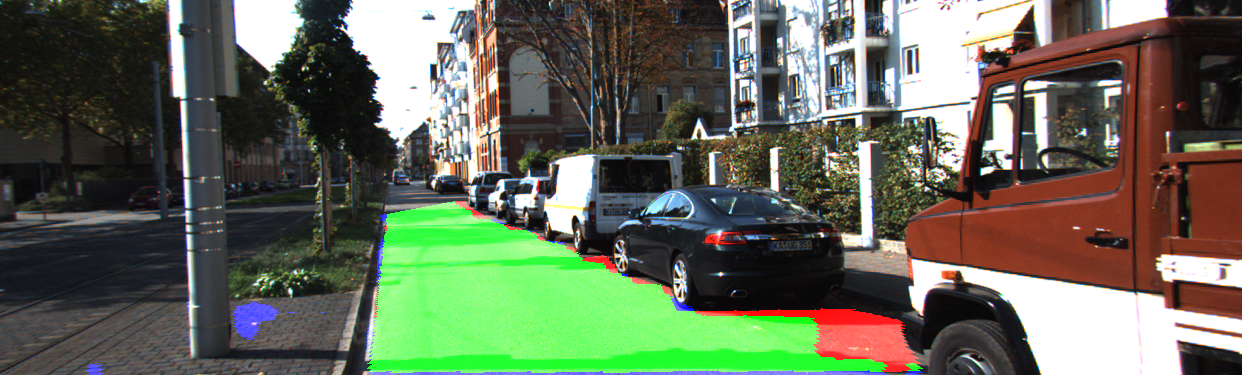This figure as: png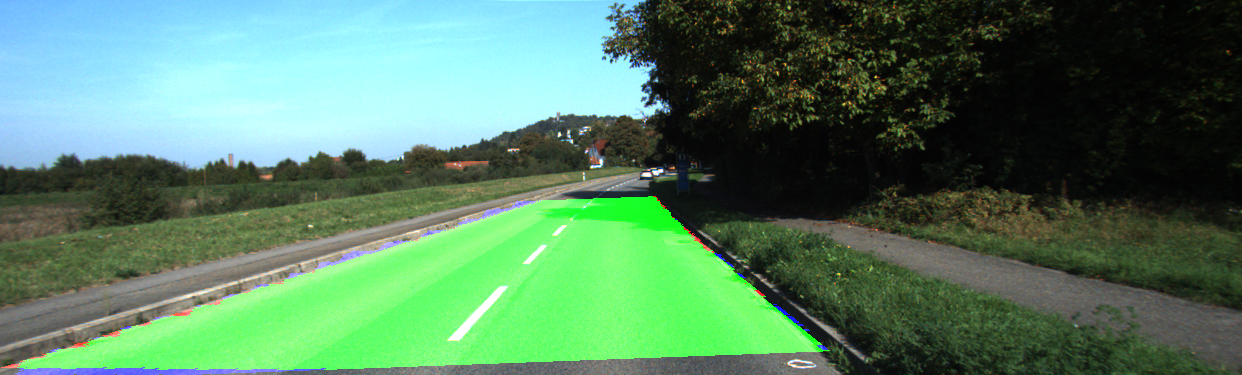This figure as: png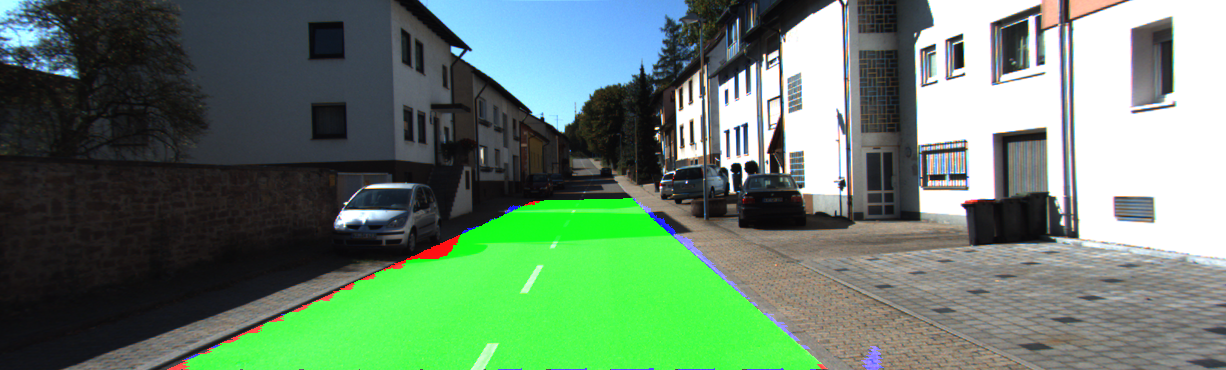This figure as: png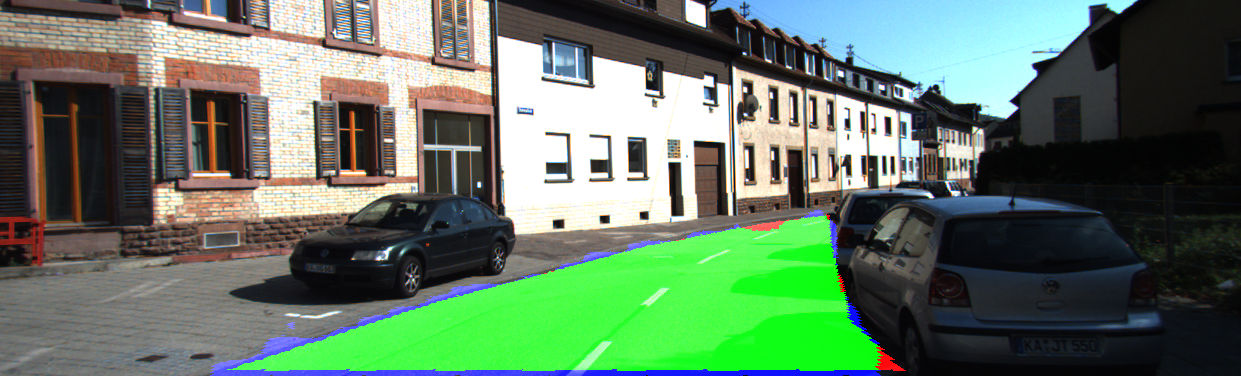This figure as: png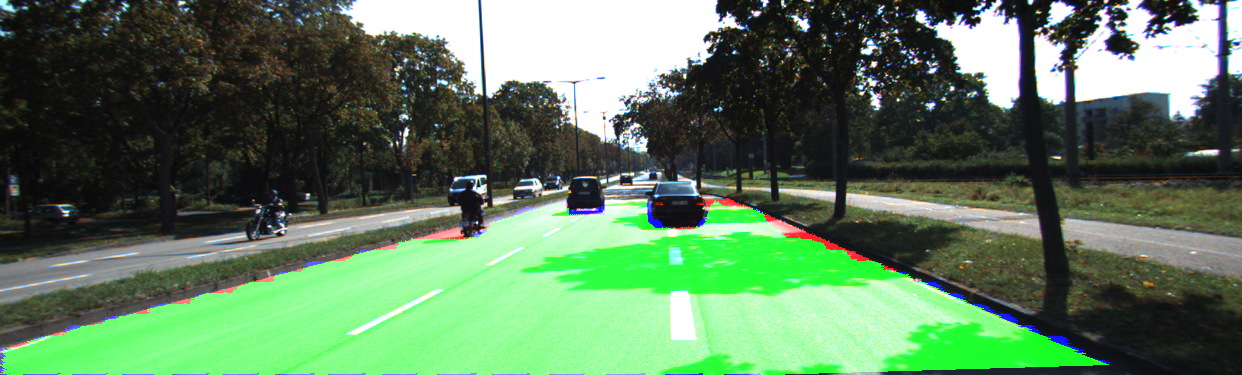This figure as: png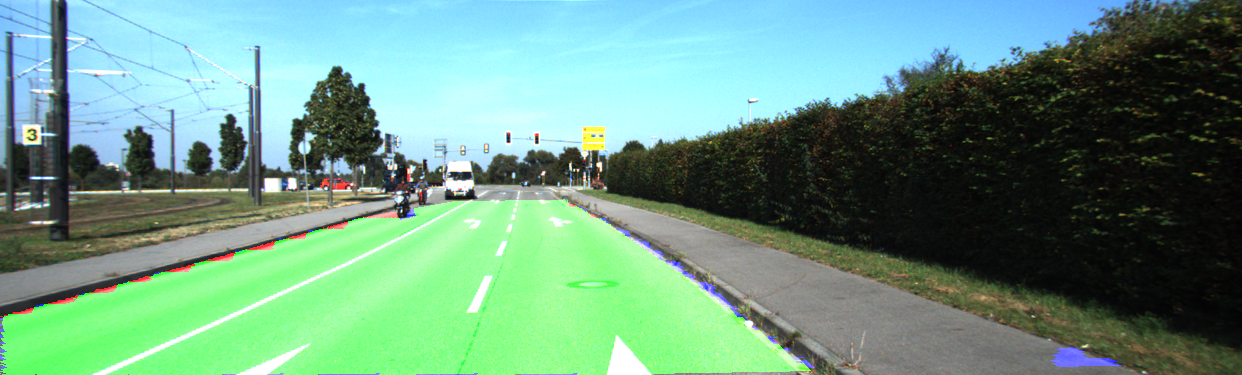This figure as: png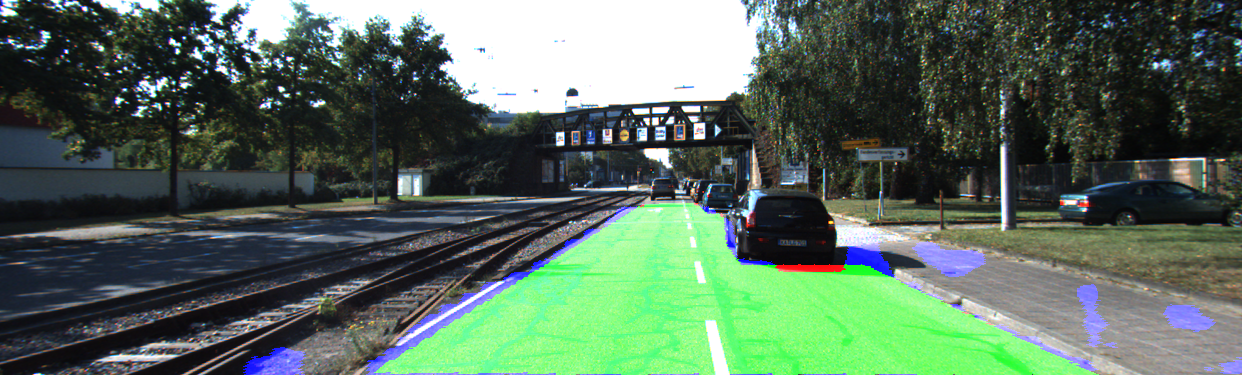This figure as: png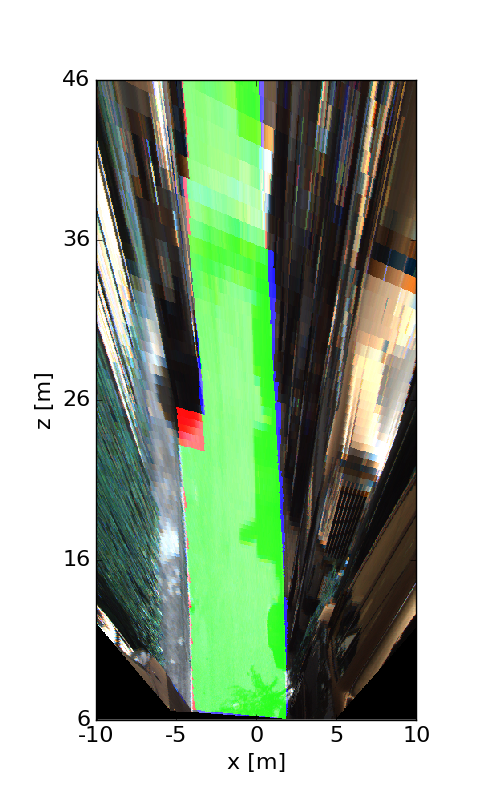This figure as: png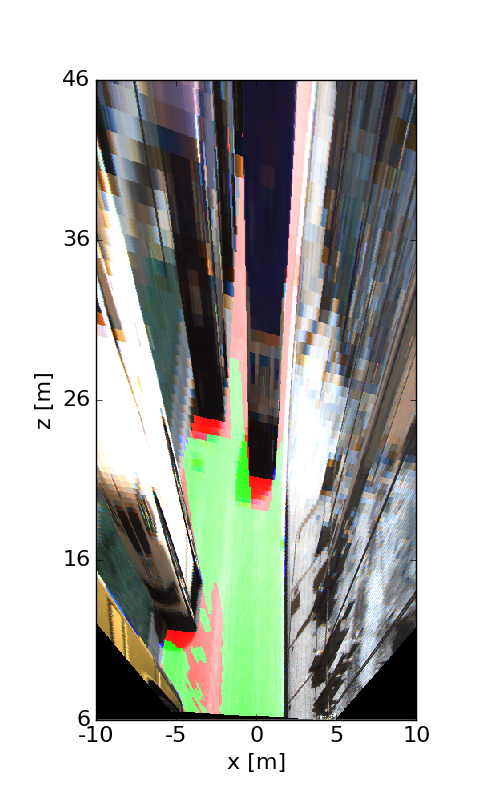This figure as: png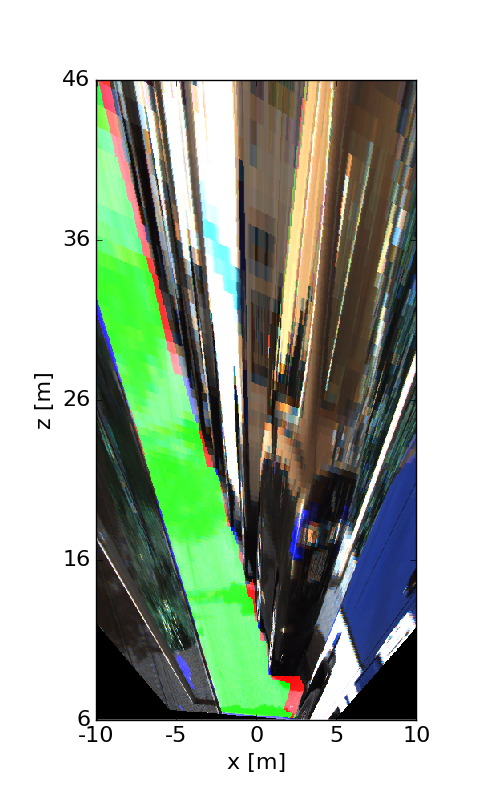This figure as: png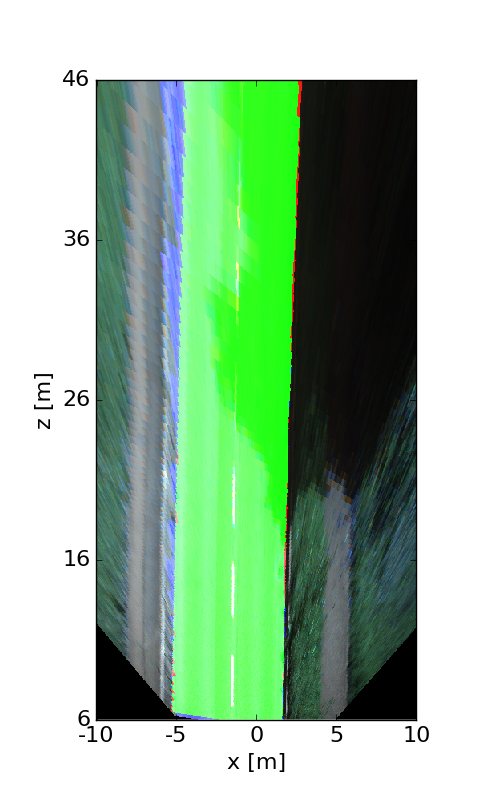This figure as: pngThis figure as: png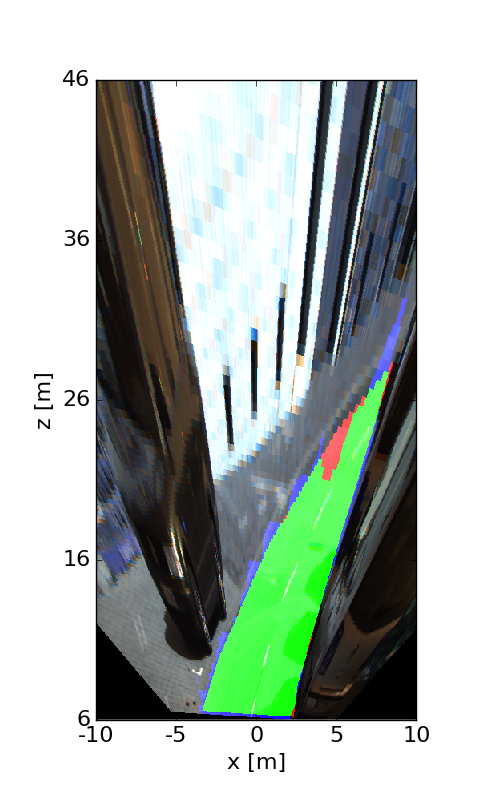This figure as: png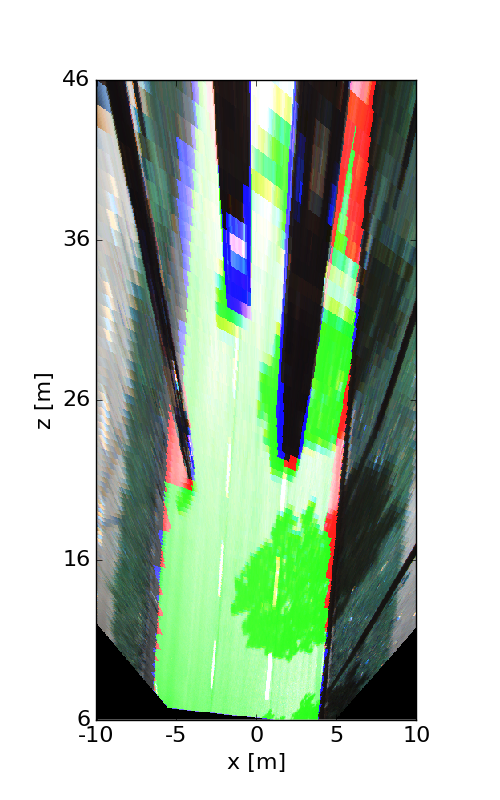This figure as: pngThis figure as: png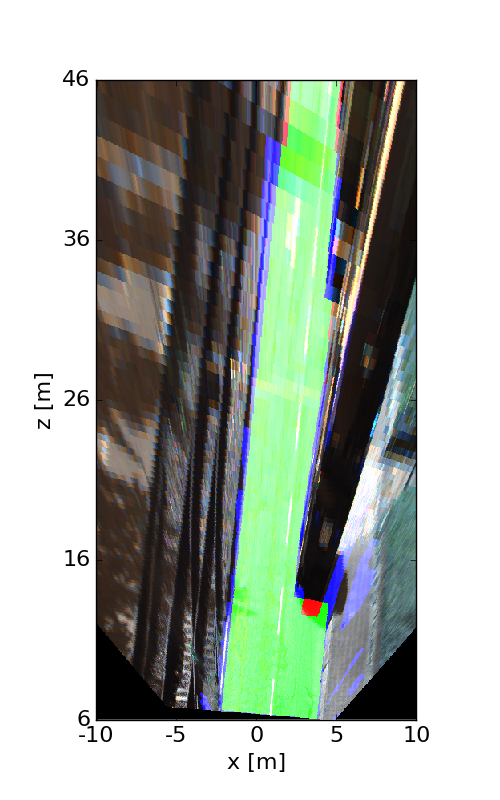This figure as: png